Silverlight能够支持的脚本目前已达10余种之多，以JavascriptIronRubyIronPython为主流。而作为HTML配偶的Javascrpit与生俱来即有对Silverlight的高度兼容，无须额外的DLL支持，相当默契。实现起来就更加简单了，仅仅一句就可轻松调用：

HtmlPage.Window.Invoke(……);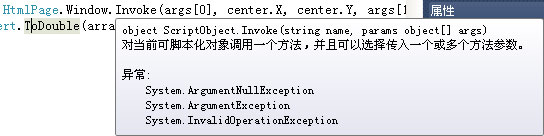Silverlight中居然有这么厉害的脚本解析方法，似乎不用它来点锦上添花感觉对不起广大读者。于是乎想到了游戏中怪物的刷新与出现，若能通过脚本编写独立的刷新位置、阵型逻辑，那么未来制作怪物、NPC的其他AI逻辑将显得游刃有余。绞尽脑汁再一次拿起被我鄙视过无数次而又迫于无奈的Javascript，吃力的编写了以下绘制各类图形的函数Script.js

//菱形路径点
function DiamondPath(centerX, centerY, arg, limit) {

var x = Math.random() * arg + centerX - arg;

var y = Math.random() * arg + centerY - arg;

return Validate(x, y, limit);
}
//圆形路径点
function RoundPath(centerX, centerY, arg, limit) {

var angle = Math.random() * 360;

var x = Math.sin(GetRadian(angle)) * arg + centerX;

var y = Math.cos(GetRadian(angle)) * arg + centerY;

return Validate(x, y, limit);
}
//正弦路径点
function SinePath(centerX, centerY, arg, limit) {

var x = Math.random() * ((4 * Math.PI) - (-4 * Math.PI)) + (-4 * Math.PI);

var y = Math.sin(x) * arg;
x
= x * arg + centerX;
y
= y + centerY;

return Validate(x, y, limit);
}
//螺旋路径点
function EddyPath(centerX, centerY, arg, limit) {

var angle = Math.random() * (360 * 4);

var x = angle * Math.cos(GetRadian(angle)) / arg + centerX;

var y = angle * Math.sin(GetRadian(angle)) / arg + centerY;

return Validate(x, y, limit);
}
//五角星路径点
function StarPath(centerX, centerY, arg, limit) {

var x, y;

var cx = 15 * arg;

var cy = 8 * arg;

var pointX = new Array(5);

var pointY = new Array(5);

for (i = 0; i < 5; i++) {

var dAngle = (i * 0.8 - 0.5* Math.PI;
pointX[i]
= cx * (0.25 + 0.24 * Math.cos(dAngle));
pointY[i]
= cy * (0.5 + 0.48 * Math.sin(dAngle));
}

var n = parseInt(Math.random() * 5);

var m = (n + 1== 5 ? 0 : (n + 1);

if (pointX[n] > pointX[m]) {
x
= Math.random() * (pointX[n] - pointX[m]) + pointX[m];
}
else {
x
= Math.random() * (pointX[m] - pointX[n]) + pointX[n];
}
y
= ((pointY[m] - pointY[n]) / (pointX[m] - pointX[n])) * (x - pointX[n]) + pointY[n] + centerY;
x
= x + centerX - cx / 5;
y
= y - cy / 2;

return Validate(x, y, limit);
}
//获取角度的弧度值

return angle * Math.PI / 180;
}
//验证x,y是否越界
function Validate(x, y, limit) {

if (x < 0) { x = 0; } else if (x > limit) { x = limit; }

if (y < 0) { y = 0; } else if (y > limit) { y = limit; }

return [x, y];
};

虽然调试时令我几近崩溃，太不友好了恨不得有空就揣上几脚，或许今后当我开发WP7游戏时才可放肆的忘掉这段伤心的往事，尔尔。

comboBox0.Items.Add(new ComboBoxItem() { Tag = "DiamondPath,10", Content = "菱形" });
new ComboBoxItem() { Tag = "RoundPath,10", Content = "圆形", IsSelected = true });
new ComboBoxItem() { Tag = "SinePath,2", Content = "正弦" });
new ComboBoxItem() { Tag = "EddyPath,100", Content = "螺旋" });
new ComboBoxItem() { Tag = "StarPath,3.33", Content = "五星" });

button4.Click
+= (s, e) => {
};

/// <summary>

/// 添加测试精灵

/// </summary>

/// <param name="num"></param>

for (int i = 0; i < num; i++) {
Sprite sprite
= new Sprite() {
......
};
......
sprite.RunRandomMoveScript(((ComboBoxItem)comboBox0.SelectedItem).Tag.ToString(), hero.Coordinate, Scene.TerrainMatrix.GetUpperBound(
0));
}
}

/// <summary>

/// 运行图形随机点移动脚本(测试调用Javascript脚本)

/// </summary>

/// <param name="arg">参数</param>

/// <param name="center">中心</param>

/// <param name="limit">边界上限</param>
public void RunRandomMoveScript(string arg, Point center, int limit) {

string[] args = arg.Split(',');

if (Application.Current.IsRunningOutOfBrowser) {
......
}
else {
Array array
= ((ScriptObject)HtmlPage.Window.Invoke(args, center.X, center.Y, args, limit)).ConvertTo<Array>();
StraightRunTo(
new Point(Convert.ToDouble(array.GetValue(0)), Convert.ToDouble(array.GetValue(1))));
}
}

够简单吧，大家可以将C#编写的所有代码当作游戏的主体逻辑，根据以上例子，我们仅需为精灵定义好一个RunRandomMoveScript方法，并在其中放上HtmlPage.Window.Invoke这句神马级的调用方法，那么前面外置的Script.js文件中的所有函数都将可以随意更改其内部逻辑而无须重新编译，更直接的好处还有当用户每次登陆网站时，他们无须再次下载那庞大的XAPDLL，浏览器会自行选择最新的js文件使用，这也是提升用户体验的良好途径，低碳且绝对环保。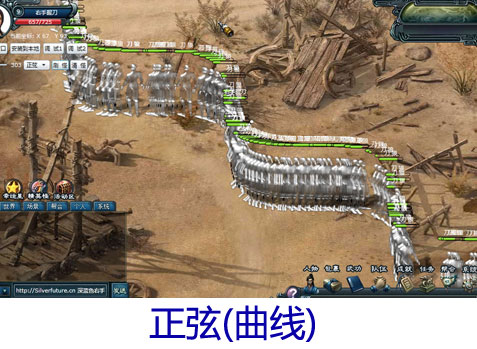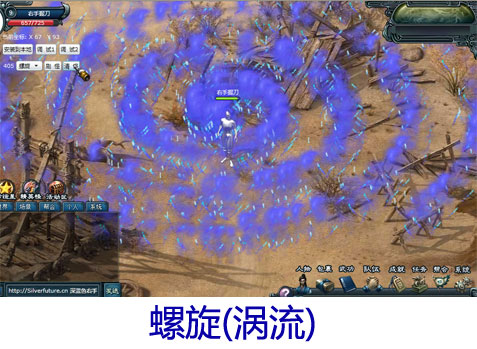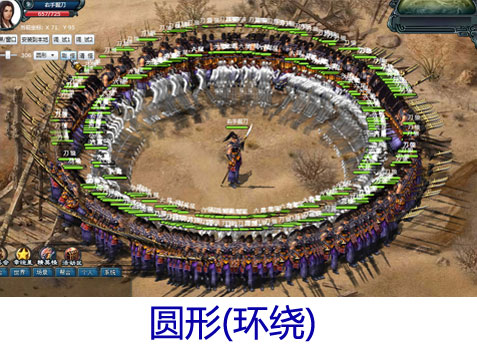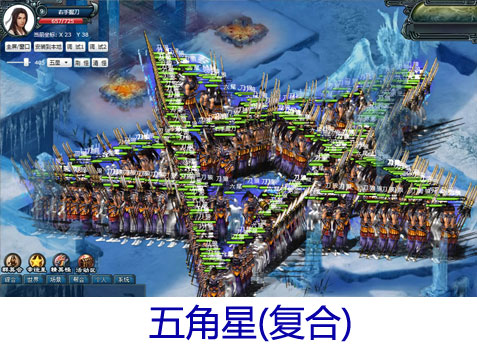/// <summary>

/// 通过命中测试获取点中的精灵们

/// </summary>
List<Sprite> HitSprite(Point p) {
List
<UIElement> hitElements = VisualTreeHelper.FindElementsInHostCoordinates(p, scene) as List<UIElement>;
List
<Sprite> hitSprites = new List<Sprite>();

for (int i = 0; i < hitElements.Count; i++) {

if (hitElements[i] is Sprite) {
as Sprite);
}
}

return hitSprites;
}

Sprite tempSprite;
//上一次命中的精灵
/// <summary>

/// 游戏中鼠标移动

/// </summary>
void LayoutRoot_MouseMove(object sender, MouseEventArgs e) {

//移动图形鼠标
Point p = e.GetPosition(this);
cursor.Coordinate
= p;

//首先判断是否命中某个精灵
List<Sprite> hitSprites = HitSprite(e.GetPosition(LayoutRoot));

bool IsHitSprite = false;

for (int i = 0; i < hitSprites.Count; i++) {

//鼠标位置为精灵有效实体区域才算命中该精灵
if (hitSprites[i].InPhysicalRange(e.GetPosition(hitSprites[i]))) {

//假如命中的精灵于前一选中精灵不同则更换选中精灵
if (tempSprite != hitSprites[i]) {

if (tempSprite != null) { tempSprite.BodyEffect = BodyEffects.None; }
tempSprite
= hitSprites[i];

//假如主角与目标阵营敌对则更换鼠标指针为攻击图形
if (hero.IsHostileTo(hitSprites[i].Camp)) {
cursor.CursorType
= CursorTypes.Attack;
hitSprites[i].BodyEffect
= BodyEffects.Red;
}
else {
cursor.CursorType
= CursorTypes.Normal;
hitSprites[i].BodyEffect
= BodyEffects.Green;
}
}
IsHitSprite
= true;

break;
}
}

//假如没有命中任何精灵则将前一选中精灵取消命中效果
if (!IsHitSprite) {

if (tempSprite != null) { tempSprite.BodyEffect = BodyEffects.None; tempSprite = null; }
cursor.CursorType
= CursorTypes.Normal;
}
}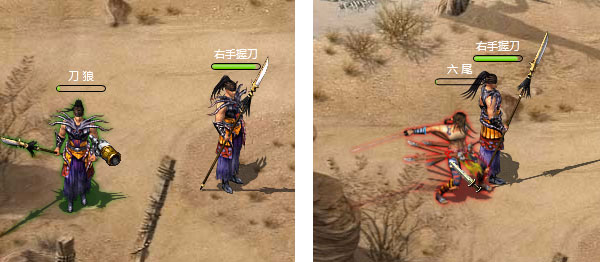posted on 2010-11-09 11:34  深蓝色右手  阅读(7291)  评论(23编辑  收藏